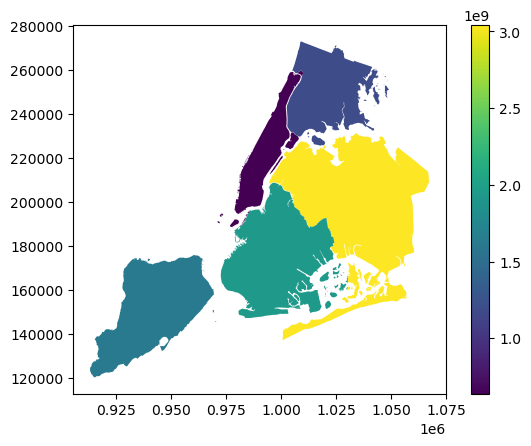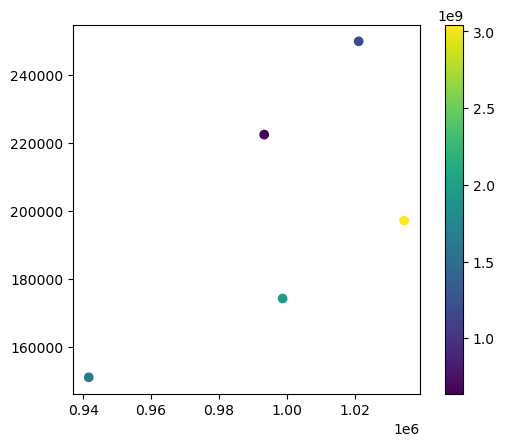# Introduction to GeoPandas¶

This quick tutorial provides an introduction to the key concepts of GeoPandas. In a few minutes, we’ll describe the basics which allow you to start your projects.

## Concepts¶

GeoPandas, as the name suggests, extends popular data science library pandas by adding support for geospatial data. If you are not familiar with pandas, we recommend taking a quick look at its Getting started documentation before proceeding.

The core data structure in GeoPandas is geopandas.GeoDataFrame, a subclass of pandas.DataFrame able to store geometry columns and perform spatial operations. Geometries are handled by geopandas.GeoSeries, a subclass of pandas.Series. Therefore, your GeoDataFrame is a combination of Series with your data (numerical, boolean, text etc.) and GeoSeries with geometries (points, polygons etc.). You can have as many columns with geometries as you wish, there’s no limit typical for desktop GIS software.Each GeoSeries can contain any geometry type (we can even mix them within a single array) and has a GeoSeries.crs attribute, which stores information on the projection (CRS stands for Coordinate Reference System). Therefore, each GeoSeries in a GeoDataFrame can be in a different projection, allowing you to have, for example, multiple versions of the same geometry, just in a different CRS.

One GeoSeries within a GeoDataFrame is seen as the active geometry, which means that all geometric operations applied to a GeoDataFrame use the specified column.

User Guide

See more on data structures in the User Guide.

Let’s see how this works in practice.

## Reading and writing files¶

First, we need to read some data.

### Read files¶

Assuming we have a file containing both data and geometry (e.g. GeoPackage, GeoJSON, Shapefile), we can easily read it using geopandas.read_file function, which automatically detects filetype and creates a GeoDataFrame. In this example, we’ll use the "nybb" dataset, a map of New York boroughs which is part of GeoPandas installation. Therefore we need to get the path to the actual file. With your file, you specify a path as a string ("my_data/my_file.geojson").

:

import geopandas

path_to_data = geopandas.datasets.get_path("nybb")
gdf = geopandas.read_file(path_to_data)

gdf

:

BoroCode BoroName Shape_Leng Shape_Area geometry
0 5 Staten Island 330470.010332 1.623820e+09 MULTIPOLYGON (((970217.022 145643.332, 970227....
1 4 Queens 896344.047763 3.045213e+09 MULTIPOLYGON (((1029606.077 156073.814, 102957...
2 3 Brooklyn 741080.523166 1.937479e+09 MULTIPOLYGON (((1021176.479 151374.797, 102100...
3 1 Manhattan 359299.096471 6.364715e+08 MULTIPOLYGON (((981219.056 188655.316, 980940....
4 2 Bronx 464392.991824 1.186925e+09 MULTIPOLYGON (((1012821.806 229228.265, 101278...

### Write files¶

Writing a GeoDataFrame back to file is similarly simple, using GeoDataFrame.to_file. The default file format is Shapefile, but you can specify your own using driver keyword.

:

gdf.to_file("my_file.geojson", driver="GeoJSON")


## Simple methods¶

Now we have our GeoDataFrame and can start working with its geometry.

Since we have only one geometry column read from the file, it is automatically seen as the active geometry and methods used on GeoDataFrame will be applied to the "geometry" column.

### Measuring area¶

To measure the area of each polygon (or MultiPolygon in this specific case), we can use GeoDataFrame.area attribute, which returns a pandas.Series. Note that GeoDataFrame.area is just GeoSeries.area applied to an active geometry column.

But first, we set the names of boroughs as an index, to make the results easier to read.

:

gdf = gdf.set_index("BoroName")

:

gdf["area"] = gdf.area
gdf["area"]

:

BoroName
Staten Island    1.623822e+09
Queens           3.045214e+09
Brooklyn         1.937478e+09
Manhattan        6.364712e+08
Bronx            1.186926e+09
Name: area, dtype: float64


### Getting polygon boundary and centroid¶

To get just the boundary of each polygon (LineString), we can call GeoDataFrame.boundary.

:

gdf['boundary'] = gdf.boundary
gdf['boundary']

:

BoroName
Staten Island    MULTILINESTRING ((970217.022 145643.332, 97022...
Queens           MULTILINESTRING ((1029606.077 156073.814, 1029...
Brooklyn         MULTILINESTRING ((1021176.479 151374.797, 1021...
Manhattan        MULTILINESTRING ((981219.056 188655.316, 98094...
Bronx            MULTILINESTRING ((1012821.806 229228.265, 1012...
Name: boundary, dtype: geometry


Since we have saved boundary as a new column, we now have two geometry columns in the same GeoDataFrame.

We can also create new geometries, which could be, for example, a buffered version of the original one (i.e., GeoDataFrame.buffer(10)) or its centroid:

:

gdf['centroid'] = gdf.centroid
gdf['centroid']

:

BoroName
Staten Island     POINT (941639.450 150931.991)
Queens           POINT (1034578.078 197116.604)
Brooklyn          POINT (998769.115 174169.761)
Manhattan         POINT (993336.965 222451.437)
Bronx            POINT (1021174.790 249937.980)
Name: centroid, dtype: geometry


### Measuring distance¶

We can also measure how far is each centroid from the first one.

:

first_point = gdf['centroid'].iloc
gdf['distance'] = gdf['centroid'].distance(first_point)
gdf['distance']

:

BoroName
Staten Island         0.000000
Queens           103781.535276
Brooklyn          61674.893421
Manhattan         88247.742789
Bronx            126996.283623
Name: distance, dtype: float64


It’s still a DataFrame, so we have all the pandas functionality available to use on the geospatial dataset, and to do data manipulations with the attributes and geometry information together.

For example, we can calculate average of the distance measured above (by accessing the 'distance' column, and calling the mean() method on it):

:

gdf['distance'].mean()

:

76140.09102166798


## Making maps¶

GeoPandas can also plot maps, so we can check how our geometries look like in space. The key method here is GeoDataFrame.plot(). In the example below, we plot the "area" we measured earlier using the active geometry column. We also want to show a legend (legend=True).

:

gdf.plot("area", legend=True)

:

<AxesSubplot:>Switching the active geometry (GeoDataFrame.set_geometry) to centroids, we can plot the same data using point geometry.

:

gdf = gdf.set_geometry("centroid")
gdf.plot("area", legend=True)

:

<AxesSubplot:>And we can also layer both GeoSeries on top of each other. We just need to use one plot as an axis for the other.

:

ax = gdf["geometry"].plot()
gdf["centroid"].plot(ax=ax, color="black")

:

<AxesSubplot:>Now we set the active geometry back to the original GeoSeries.

:

gdf = gdf.set_geometry("geometry")


User Guide

See more on mapping in the User Guide.

## Geometry creation¶

We can further work with the geometry and create new shapes based on those we already have.

### Convex hull¶

If we are interested in the convex hull of our polygons, we can call GeoDataFrame.convex_hull.

:

gdf["convex_hull"] = gdf.convex_hull

:

ax = gdf["convex_hull"].plot(alpha=.5)  # saving the first plot as an axis and setting alpha (transparency) to 0.5
gdf["boundary"].plot(ax=ax, color="white", linewidth=.5)  # passing the first plot and setting linewitdth to 0.5

:

<AxesSubplot:>### Buffer¶

In other cases, we may need to buffer the geometry using GeoDataFrame.buffer(). Geometry methods are automatically applied to the active geometry, but we can apply them directly to any GeoSeries as well. Let’s buffer the boroughs and their centroids and plot both on top of each other.

:

# buffering the active geometry by 10 000 feet (geometry is already in feet)
gdf["buffered"] = gdf.buffer(10000)

# buffering the centroid geometry by 10 000 feet (geometry is already in feet)
gdf["buffered_centroid"] = gdf["centroid"].buffer(10000)

:

ax = gdf["buffered"].plot(alpha=.5)  # saving the first plot as an axis and setting alpha (transparency) to 0.5
gdf["buffered_centroid"].plot(ax=ax, color="red", alpha=.5)  # passing the first plot as an axis to the second
gdf["boundary"].plot(ax=ax, color="white", linewidth=.5)  # passing the first plot and setting linewitdth to 0.5

:

<AxesSubplot:>User Guide

## Geometry relations¶

We can also ask about the spatial relations of different geometries. Using the geometries above, we can check which of the buffered boroughs intersect the original geometry of Brooklyn, i.e., is within 10 000 feet from Brooklyn.

First, we get a polygon of Brooklyn.

:

brooklyn = gdf.loc["Brooklyn", "geometry"]
brooklyn

:The polygon is a shapely geometry object, as any other geometry used in GeoPandas.

:

type(brooklyn)

:

shapely.geometry.multipolygon.MultiPolygon


Then we can check which of the geometries in gdf["buffered"] intersects it.

:

gdf["buffered"].intersects(brooklyn)

:

BoroName
Staten Island     True
Queens            True
Brooklyn          True
Manhattan         True
Bronx            False
dtype: bool


Only Bronx (on the north) is more than 10 000 feet away from Brooklyn. All the others are closer and intersect our polygon.

Alternatively, we can check which buffered centroids are entirely within the original boroughs polygons. In this case, both GeoSeries are aligned, and the check is performed for each row.

:

gdf["within"] = gdf["buffered_centroid"].within(gdf)
gdf["within"]

:

BoroName
Staten Island     True
Queens            True
Brooklyn         False
Manhattan        False
Bronx            False
Name: within, dtype: bool


We can plot the results on the map to confirm the finding.

:

gdf = gdf.set_geometry("buffered_centroid")
ax = gdf.plot("within", legend=True, categorical=True, legend_kwds={'loc': "upper left"})  # using categorical plot and setting the position of the legend
gdf["boundary"].plot(ax=ax, color="black", linewidth=.5)  # passing the first plot and setting linewitdth to 0.5

:

<AxesSubplot:>## Projections¶

Each GeoSeries has the Coordinate Reference System (CRS) accessible as GeoSeries.crs. CRS tells GeoPandas where the coordinates of geometries are located on the Earth. In some cases, CRS is geographic, which means that coordinates are in latitude and longitude. In those cases, its CRS is WGS84, with the authority code EPSG:4326. Let’s see the projection of our NY boroughs GeoDataFrame.

:

gdf.crs

:

<Projected CRS: EPSG:2263>
Name: NAD83 / New York Long Island (ftUS)
Axis Info [cartesian]:
- X[east]: Easting (US survey foot)
- Y[north]: Northing (US survey foot)
Area of Use:
- name: United States (USA) - New York - counties of Bronx; Kings; Nassau; New York; Queens; Richmond; Suffolk.
- bounds: (-74.26, 40.47, -71.8, 41.3)
Coordinate Operation:
- name: SPCS83 New York Long Island zone (US Survey feet)
- method: Lambert Conic Conformal (2SP)
Datum: North American Datum 1983
- Ellipsoid: GRS 1980
- Prime Meridian: Greenwich


Geometries are in EPSG:2263 with coordinates in feet. We can easily re-project a GeoSeries to another CRS, like EPSG:4326 using GeoSeries.to_crs().

:

gdf = gdf.set_geometry("geometry")
boroughs_4326 = gdf.to_crs("EPSG:4326")
boroughs_4326.plot()

:

<AxesSubplot:>:

boroughs_4326.crs

:

<Geographic 2D CRS: EPSG:4326>
Name: WGS 84
Axis Info [ellipsoidal]:
- Lat[north]: Geodetic latitude (degree)
- Lon[east]: Geodetic longitude (degree)
Area of Use:
- name: World.
- bounds: (-180.0, -90.0, 180.0, 90.0)
Datum: World Geodetic System 1984
- Ellipsoid: WGS 84
- Prime Meridian: Greenwich


Notice the difference in coordinates along the axes of the plot. Where we had 120 000 - 280 000 (feet) before, we have 40.5 - 40.9 (degrees) now. In this case, boroughs_4326 has a "geometry" column in WGS84 but all the other (with centroids etc.) remains in the original CRS.

Warning

For operations that rely on distance or area, you always need to use projected CRS (in meters, feet, kilometers etc.) not a geographic one. GeoPandas operations are planar, and degrees reflect the position on a sphere. Therefore the results may not be correct. For example, the result of gdf.area.sum() (projected CRS) is 8 429 911 572 ft2 but the result of boroughs_4326.area.sum() (geographic CRS) is 0.083.

User Guide

See more on projections in the User Guide.

## What next?¶

With GeoPandas we can do much more that this, from aggregations, to spatial joins, geocoding and much more.

Head to the User Guide for to learn more about different functionality of GeoPandas, to the Examples to see how it can be used or the the API reference for the details.

[ ]: